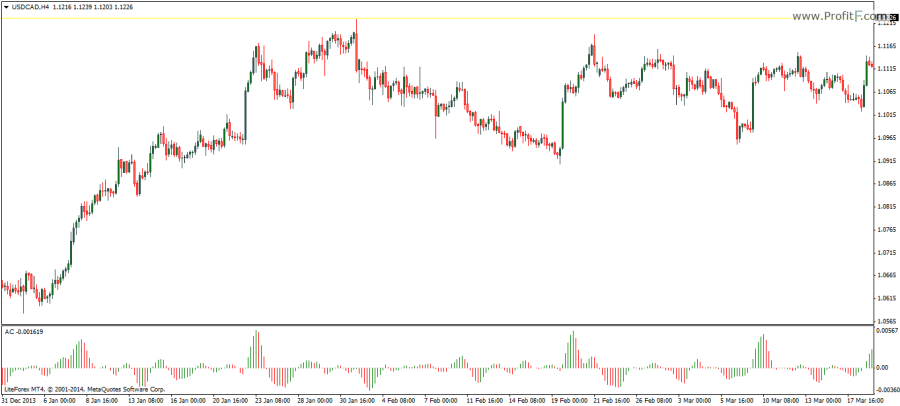# Acceleration/Deceleration indicator

The Acceleration/Deceleration indicator (AC) is used to measure the rate of change or the acceleration or deceleration of the market prices. It is commonly known as the AC indicator for short. This oscillator changes direction even before the underlying forces in the market change. The AC oscillator therefore can be used as an early warning indicator of possible price changes or market turns.The Acceleration/Deceleration oscillator is plotted as histogram and oscillates above and below the 0-line. The Acceleration/Deceleration oscillator calculates the difference between the 5 and 34 period simple moving average applied to the median price (median price = High+Low/2). The value is then subtracted from the Accelerator Oscillator or AO for the 5 periods.

When prices start to lose momentum, the AC oscillator first signals this by changing its bar from Green to Red, while still above the 0-line. A sell signal is triggered when the AC oscillator turns below the 0-line and is Red. When the AC oscillator turns to green, while still below the 0-line, it signals an early warning of a change in price direction. A buy signal is triggered with the AC oscillator turns above the 0-line.

Besides the 0-line crossing, divergences can also be traded with the AC oscillator although it is not that common.

Rate this post:(No Ratings Yet)Loading...
---